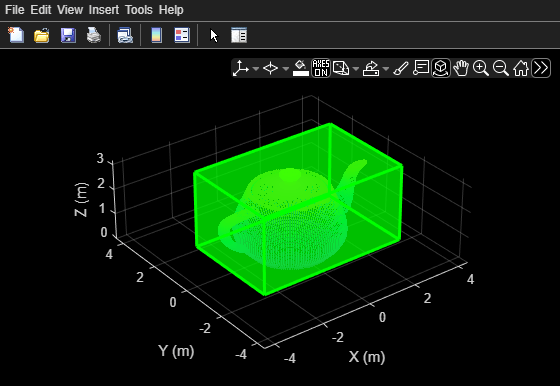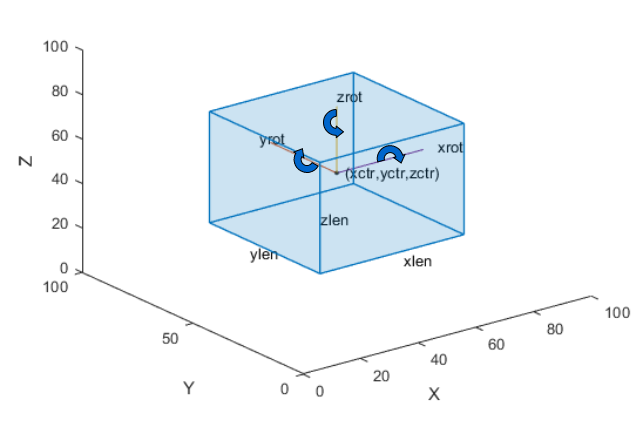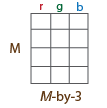# showShape

Display shapes on image, video, or point cloud

Since R2020b

## Syntax

``showShape(shape,position)``
``showShape(___,Name=Value)``

## Description

example

````showShape(shape,position)` displays one or more instances of a shape `shape` in the current axes at the specified locations `position`.```

example

````showShape(___,Name=Value)` specifies options using one or more name-value arguments in addition to the input arguments from the previous syntax. For example, `Color="yellow"` sets the color for the shapes to yellow.```

## Examples

collapse all

Read an image into the workspace.

`I = imread("visionteam1.jpg");`

Create an aggregate channel features (ACF) people detector.

`detector = peopleDetectorACF`
```detector = acfObjectDetector with properties: ModelName: 'inria-100x41' ObjectTrainingSize: [100 41] NumWeakLearners: 2048 ```

Detect people in the image.

`[bboxes,scores] = detect(detector,I);`

Display the image with a labeled rectangle around each detected person. Display the associated detection score around each detected person.

```figure imshow(I) labels = "person:" + scores; showShape("rectangle",bboxes,Label=labels)```Read point cloud data into the workspace.

`ptCloud = pcread("teapot.ply");`

Display the point cloud data.

```figure pcshow(ptCloud) xlabel("X") ylabel("Y") zlabel("Z")```

Define a cuboid and display it in green with an opacity of `0.5`.

```pos = [0.3753 0 1.65 6 4 3 0 0 0]; showShape("cuboid",pos,Color="green",Opacity=0.5)```Read point cloud data into the workspace.

`ptCloud = pcread("teapot.ply");`

Define a rotation matrix and 3-D transform to rotate the point cloud, and an associated cuboid, by 5 degrees.

```rot = 5; R = [ cosd(rot) sind(rot) 0 0; ... -sind(rot) cosd(rot) 0 0; ... 0 0 1 0; ... 0 0 0 1]; tform = affine3d(R);```

Compute the x and y rendered limits of the point cloud, to ensure that you do not clip the point cloud during rotation.

```pcLimits = abs([ptCloud.XLimits ptCloud.YLimits]); maxLimit = max(pcLimits);```

Add a margin to the plot to prevent the rotation from clipping the cuboid.

```margin = 1; maxLimit = maxLimit + margin; xlimits = [-maxLimit maxLimit]; ylimits = [-maxLimit maxLimit]; zlimits = ptCloud.ZLimits;```

Create a player for visualizing the point cloud.

`player = pcplayer(xlimits,ylimits,zlimits);`

Customize the player axes labels.

```xlabel(player.Axes,"X (m)"); ylabel(player.Axes,"Y (m)"); zlabel(player.Axes,"Z (m)");```

Define a cuboid around the point cloud.

`cuboidPosition = [0.3753 0 1.65 6 4 3 0 0 0];`

Define the output view for a cuboid rotation. Use the same limits as the player so that the view does not clip the cuboid. Then, display the rotated point cloud and cuboid.

```gridSize = [1 1 1]; ref = imref3d(gridSize,xlimits,ylimits,zlimits); for i = 1:round((360/rot)) % Rotate the point cloud. ptCloud = pctransform(ptCloud,tform); % Rotate the cuboid. cuboidPosition = bboxwarp(cuboidPosition,tform,ref); % Show the rotated point cloud data. view(player,ptCloud) % Show the rotated cuboid. showShape("cuboid",cuboidPosition, ... Parent=player.Axes, ... Color="green", ... Opacity=0.5) % Use drawnow to synchronize the point cloud and shape visualizations. drawnow end```## Input Arguments

collapse all

Type of shape, specified as `"rectangle"`, `"filled-rectangle"`, `"line"`, `"polygon"`, `"filled-polygon"`, `"circle"`, `"filled-circle"`, or `"projected-cuboid"`.

Data Types: `char`

Position of shape, specified according to the type of shape, as described in the table.

ShapePositionExample
`rectangle`

For one or more axis-aligned rectangles or filled rectangles, specify as an M-by-4 numeric matrix, where each row specifies a rectangle of the form $\left[\begin{array}{cccc}x& y& width& height\end{array}\right]$.

• M is the number of axis-aligned rectangles.

• x and `y` specify the upper-left corner of the rectangle.

• w specifies the width of the rectangle, which is its length along the x-axis.

• h specifies the height of the rectangle, which is its length along the y-axis.

`$\left[\begin{array}{cccc}{x}_{1}& {y}_{1}& widt{h}_{1}& heigh{t}_{1}\\ {x}_{2}& {y}_{2}& widt{h}_{2}& heigh{t}_{2}\\ ⋮& ⋮& ⋮& ⋮\\ {x}_{M}& {y}_{M}& widt{h}_{M}& heigh{t}_{M}\end{array}\right]$`For one or more rotated rectangles, specify in spatial coordinates as an M-by-5 numeric matrix, where each row specifies a rotated rectangle of the form [xctr yctr w h yaw].

• M is the number of rotated rectangles.

• xctr and yctr specify the center of the rectangle.

• w specifies the width of the rectangle, which is its length along the x-axis before rotation.

• h specifies the height of the rectangle, which is its length along the y-axis before rotation.

• yaw specifies the rotation angle in degrees. The rotation is clockwise-positive around the center of the rectangle.`cuboid`

For one or more cuboids, specify in spatial coordinates as an M-by-9 numeric matrix where each row specifies a cuboid of the form [xctr yctr zctr xlen ylen zlen xrot yrot zrot], where

• M is the number of cuboids.

• xctr, yctr, and zctr specify the center of the cuboid.

• xlen, ylen, and zlen specify the length of the cuboid along the x-axis, y-axis, and z-axis, respectively, before rotation.

• xrot, yrot, and zrot specify the rotation angles of the cuboid around the x-axis, y-axis, and z-axis, respectively. The xrot, yrot, and zrot rotation angles are in degrees about the cuboid center. Each rotation is clockwise-positive with respect to the positive direction of the associated spatial axis. The function computes rotation matrices assuming `ZYX` order Euler angles [xrot yrot zrot].`circle`

For one or more circles, specify spatial coordinates as an M-by-3 numeric matrix, where each row specifies a circle of the form [xctr yctr radius].

• M is the number of circles.

• xctr and yctr specify the center of the circle.

`$\left[\begin{array}{ccc}xct{r}_{1}& yct{r}_{1}& radiu{s}_{1}\\ xct{r}_{2}& yct{r}_{2}& radiu{s}_{2}\\ ⋮& ⋮& ⋮\\ xct{r}_{M}& yct{r}_{M}& radiu{s}_{M}\end{array}\right]$``line`

For one or more lines, specify spatial coordinates using one of these formats to specify a single line with connected endpoints, multiple lines with the same number of endpoints, or multiple lines with different numbers of endpoints.

• Single lines with connected endpoints — Specify as a P-by-2 matrix, where each row specifies an endpoint in the form [x y], and the endpoint in one row connects directly to the endpoint in the next. P is the number of endpoints in the line.

• Multiple lines with the same number of endpoints — Specify as an M-by-2P matrix, where each row specifies the consecutive endpoints of a single line in the form [x1 y1 x2 y2 ... xp yp]. Each [x y] pair is the coordinates of a single endpoint. M is the number of specified lines, and P is the number of endpoints per line.

• Multiple lines with different numbers of endpoints — Specify as an M-by-1 cell array, where each cell contains the endpoints for a single line as a P-by-2 matrix or a 1-by-2P vector]. M is the number of specified lines, and P is the number of endpoints for each line`polygon`

For one or more polygons, specify in spatial coordinates as an M-by-1 cell array, where each cell contains an L-by-2 matrix of [x y] vertex locations or a 1-by-2L vector of consecutive vertex locations of the form [x1, y1, x2,y2, … xL,yL ].

• M is the number of polygons.

• Each [x y] pair describes a vertex location or endpoint.

• L is the number of vertices in a polygon or endpoints in a line. Each polygon or line can have a different number of vertices or endpoints.` projected-cuboid`

For one or more projected cuboids, specify in spatial coordinates as an 8-by-2-by-M array or an M-by-8 matrix, where M is the number of projected cuboids.

When specified as an 8-by-2-by-M array, each row must contain the [x y] location of a projected cuboid vertex. The vertices connect to form a cuboid with six faces. The order of the input vertices must match the order shown in the diagram.

When specified as an M-by-8 matrix, each row specifies the dimensions of the front-facing and rear-facing sides of a projected cuboid in the form, [x1 y1 w1 h1 x2 y2 w2 h2], where [x1 y1] and [x2 y2] specify the upper-left coordinates of the front-facing and rear-facing sides, respectively, and [w1 h1] and [w2 h2] specify the corresponding widths and heights.### Name-Value Arguments

Specify optional pairs of arguments as `Name1=Value1,...,NameN=ValueN`, where `Name` is the argument name and `Value` is the corresponding value. Name-value arguments must appear after other arguments, but the order of the pairs does not matter.

Example: ` showShape("rectangle",bboxes,Color="yellow")` sets the color for the shapes to yellow.

Before R2021a, use commas to separate each name and value, and enclose `Name` in quotes.

Example: ` showShape("rectangle",bboxes,"Color","yellow")` sets the color for the shapes to yellow.

Shape label, specified as a scalar, M-element vector, or an M-element cell array of character vectors, where M is the number of shapes.

If the input is a scalar value, then the function applies the label to each shape. If the input is an M-element vector or cell array, then the function applies the unique label to the corresponding shape, using the order in which you specify the shapes to the function.

Shape color, specified as a short color name, color name, vector of color names, three-column matrix of RGB triplets.

The supported colors table lists RGB intensities in the range [0, 1], but you must specify RGB triplets in the range of your selected data type. For example, if specifying this argument as a matrix of `uint8` values, you must convert each intensity value to the range [0, 255]. To convert the listed intensity values to a `uint8` data type, use the code `uint8(255*intensity)`, where intensity is an RGB triplet value listed in the table.

You can specify a different color for each shape or one color for all shapes. To specify one color for all markers, specify `Color` as a color name or an [R G B] vector.

SpecificationFormatExample
Specify one color for all shapes (or markers)

Short color name or color name

`"r"`

`"red"`

RGB triplet

`[1 0 0]`Specify a color for each shape (or marker)

Vector of color names

`["red","yellow","blue"]`

Three-column matrix of RGB triplets

```[1 0 0 0 1 1 1 0 1 1 1 1]```This table lists the supported shape colors.

Color NameShort NameRGB TripletAppearance
`"red"``"r"``[1 0 0]``"green"``"g"``[0 1 0]``"blue"``"b"``[0 0 1]``"cyan"` `"c"``[0 1 1]``"magenta"``"m"``[1 0 1]``"yellow"``"y"``[1 1 0]``"black"``"k"``[0 0 0]``"white"``"w"``[1 1 1]`Data Types: `logical` | `uint8` | `uint16` | `int16` | `double` | `single` | `cell`

Opacity of the shape fill, specified as a scalar or M-element vector of numeric values in the range [0 1], where M is the number of shapes. To use the same opacity for all shapes, specify a scalar opacity value. For a fully opaque shape fill, specify `Opacity` as `1`.

Output axes, specified as an `Axes` graphics object. For more information on Axes object properties, see Axes Properties.

Shape border line color, specified as a short color name, color name, vector of color names, three-column matrix of RGB triplets.

The supported colors table lists RGB intensities in the range [0, 1], but you must specify RGB triplets in the range of your selected data type. For example, if specifying this argument as a matrix of `uint8` values, you must convert each intensity value to the range [0, 255]. To convert the listed intensity values to a `uint8` data type, use the code `uint8(255*intensity)`, where intensity is an RGB triplet value listed in the table.

You can specify a different color for each shape or one color for all shapes. To specify one color for all markers, specify `LineColor` as a color name or an [R G B] vector.

Border line width in pixels, specified as a positive integer or an M-element vector of positive integers, where M is the number of shapes. To use the same line width for all shapes, specify a scalar positive integer. Otherwise, specify an M-element vector of positive integers.

Border line opacity of shape, specified as a scalar or M-element vector of numeric values in the range [0 1], where M is the number of shapes. To use the same opacity for all shapes, specify a scalar opacity value. For a fully opaque shape fill, specify `Opacity` as `1`.

Label text color, specified as a short color name, color name, vector of color names, three-column matrix of RGB triplets.

The supported colors table lists RGB intensities in the range [0, 1], but you must specify RGB triplets in the range of your selected data type. For example, if specifying this argument as a matrix of `uint8` values, you must convert each intensity value to the range [0, 255]. To convert the listed intensity values to a `uint8` data type, use the code `uint8(255*intensity)`, where intensity is an RGB triplet value listed in the table.

You can specify a different color for each label or one color for all labels. To specify one color for all markers, specify `LabelTextColor` as a color name or an [R G B] vector.

Label opacity of shape, specified as a scalar or M-element vector of numeric values in the range [0 1], where M is the number of shapes. To use the same opacity for all labels, specify a scalar opacity value. For a fully opaque shape fill, specify `Opacity` as `1`.

Label font, specified as `"FixedWidth"` or a font that your system supports. To display and print text properly, you must select a font that your system supports. If you select an unsupported font, the function returns an error. If you specify `"FixedWidth"`, the function uses the font specified by the `FixedWidthFont` property of the Root Properties object. The fixed-width font relies on the value of `get(0,"FixedWidthFontName")`.

Label font size in points, specified as a positive scalar.

Original orientation indicator, specified as a numeric or logical `1` (`true`) or `0` (`false`). A value of `1` (`true`) displays the original orientation of the rotated rectangle by using an arrow annotation on the bounding box. The original orientation is defined as a zero rotation angle. A value of `0` (`false`) does not show the arrow annotation. The `ShowOrientation` name-value argument applies only to rotated rectangles.

Data Types: `logical` | `integer`

## Version History

Introduced in R2020b

expand all